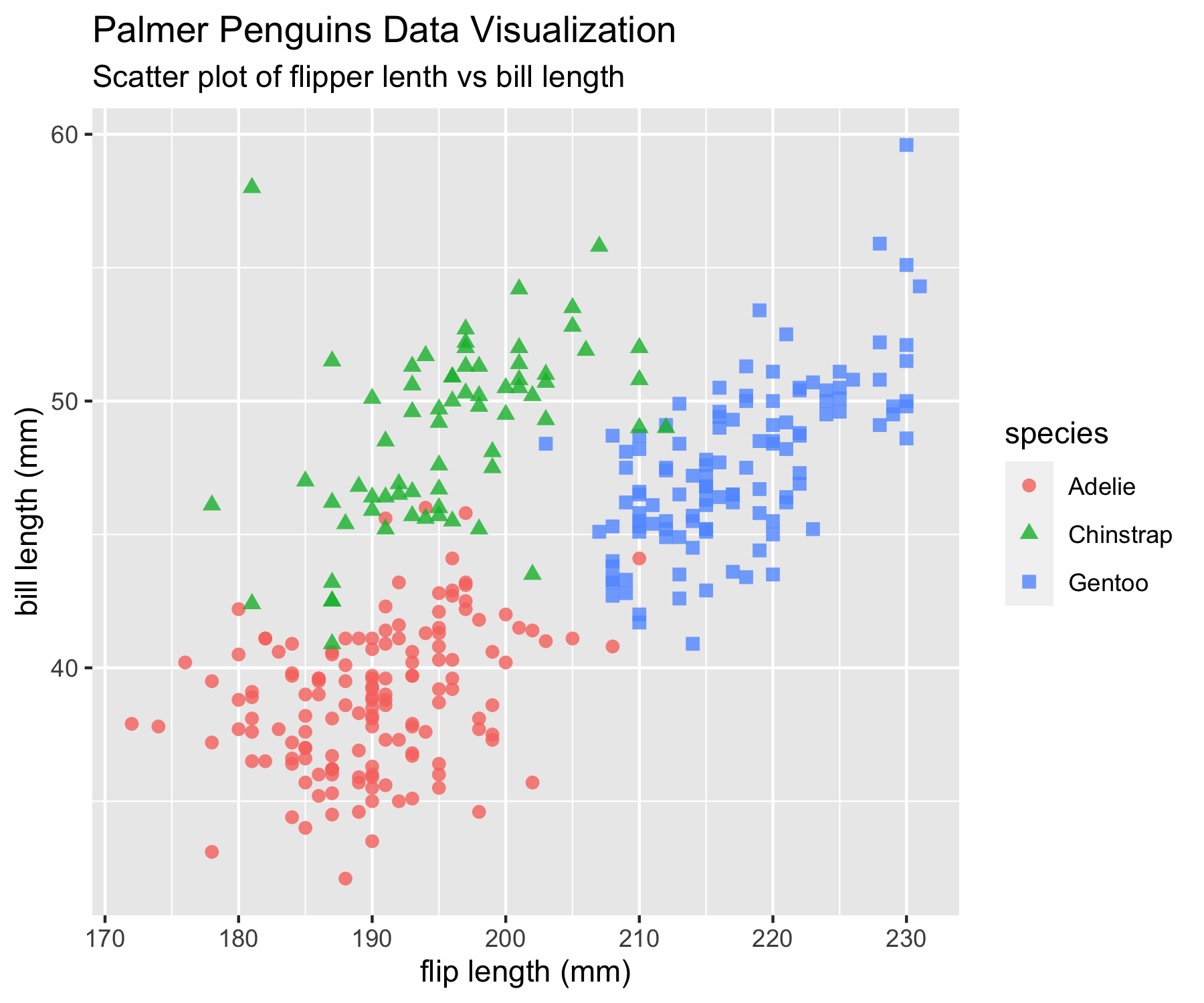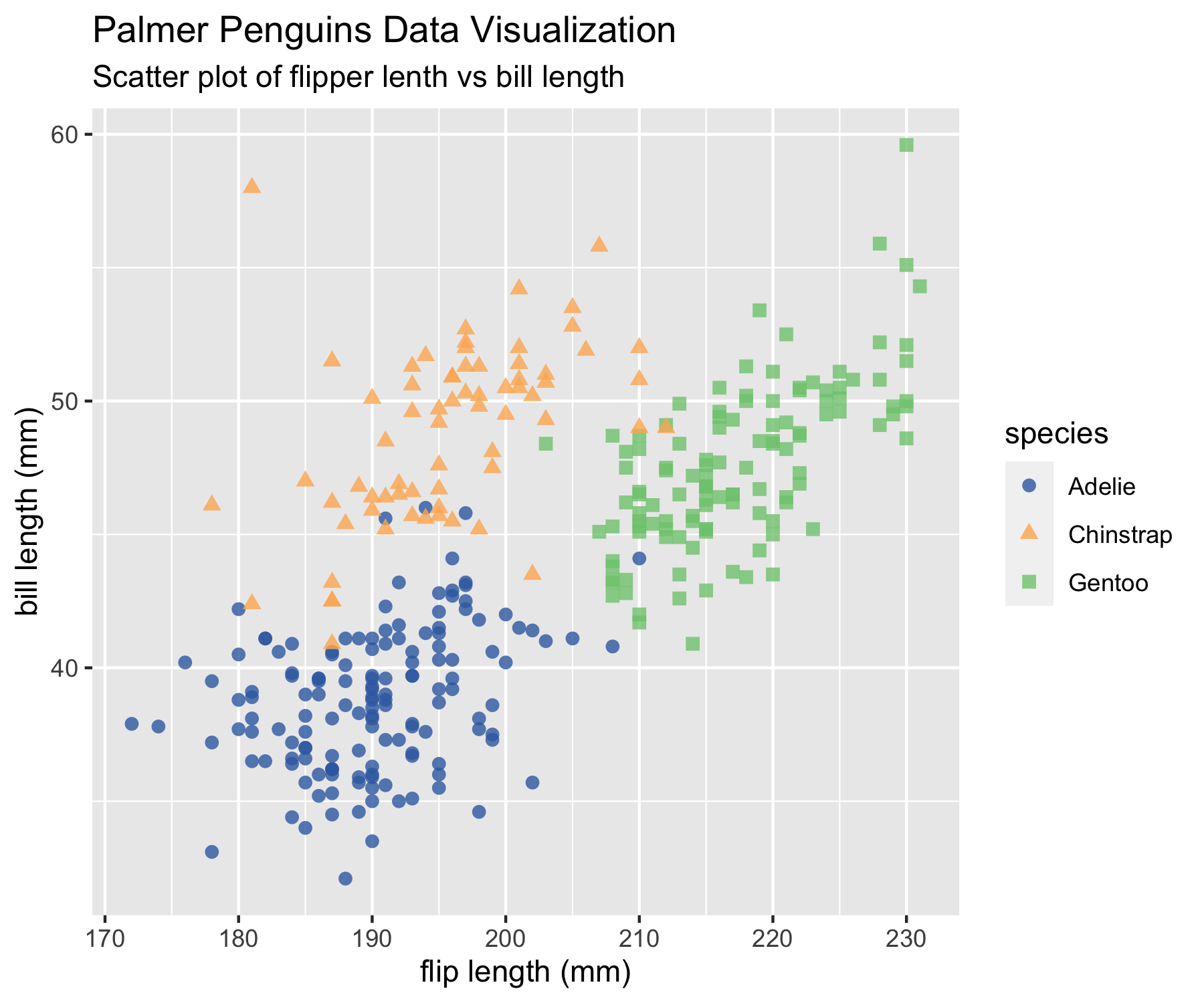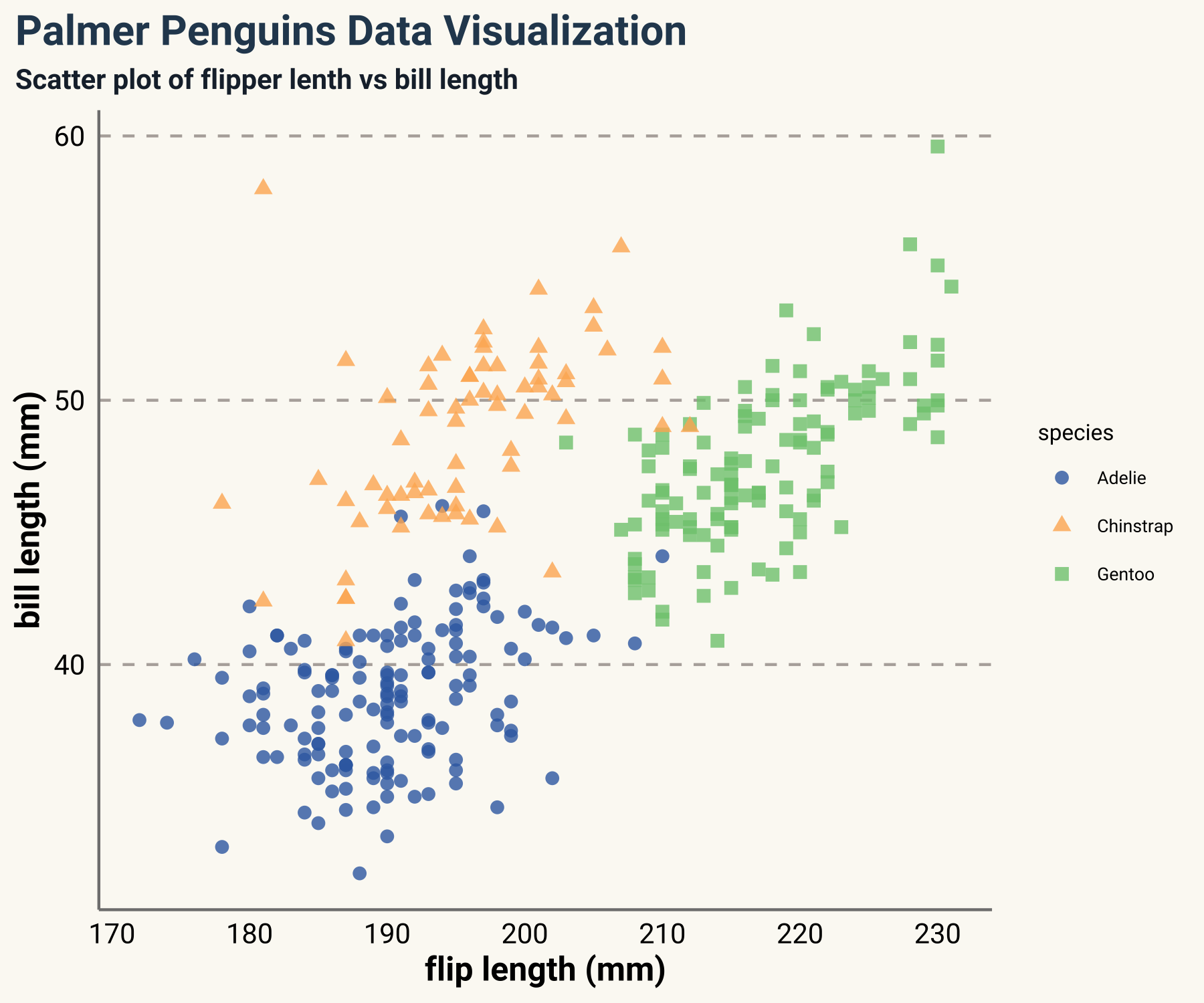In this tutorial, I summarized some useful websites to help us dealing with themes and colors in `ggplot2`.

`ggplot2` allows colors with predefined names and with hex code (e.g.: “#FF0000”). Some predefined color names can be found here:

Besides, I like exploring other color palettes from the following two websites:

Fortunately, some data visualization enthusiasts create some user-friendly R packages with a wide range of many beautiful themes and color palettes.

If you have patience and you can actually create your own themes and color palettes. The official document about modifying `ggplot` theme can be found here

For example, here is my most frequently used theme set up.

``````library(showtext)
library(ggplot2)

showtext_auto()

theme_set(theme_bw())
theme_update(
text = element_text(family = "roboto", size = 8, color = "black"),
plot.title = element_text(family = "roboto", size = 15,
face = "bold", color="#2a475e"),
plot.subtitle = element_text(family = "roboto", size = 10,
face = "bold", color="#1b2838"),
plot.caption = element_text(size = 10),
axis.title.y = element_text(face="bold", angle=90),
axis.title.x = element_text(face="bold"),
axis.text.x = element_text(size = 10, color = "black"),
axis.text.y = element_text(size = 10, color = "black"),
axis.title = element_text(size=12),
rect = element_blank(),
panel.grid = element_line(color = "#b4aea9"),
panel.grid.minor = element_blank(),
panel.grid.major.x = element_blank(),
plot.title.position = "plot",
panel.grid.major.y = element_line(linetype="dashed"),
axis.ticks = element_blank(),
axis.line = element_line(colour = "grey50"),
plot.background = element_rect(fill = '#fbf9f4', color = '#fbf9f4')
)
``````

You can also create your own color palettes:

``````library(scales)
library(ggplot2)

scale_fill_tuo <- function(...){
ggplot2::discrete_scale(
"fill","tuo",
scales::manual_pal(
values = c(
"#386cb0","#fdb462","#7fc97f",
"#ef3b2c","#662506","#a6cee3",
"#fb9a99","#984ea3","#ffff33", "#000099")),
...)
}

scale_color_tuo <- function(...){
ggplot2::discrete_scale(
"colour","tuo",
scales::manual_pal(
values = c(
"#386cb0","#fdb462","#7fc97f",
"#ef3b2c","#662506","#a6cee3",
"#fb9a99","#984ea3","#ffff33","#000099")),
...)
}
``````
``````library(tidyverse)
library(palmerpenguins)

penguins_comp <- penguins %>%
drop_na()

penguins_comp %>%
ggplot(aes(x=flipper_length_mm, y=bill_length_mm)) +
geom_point(aes(color=species, shape=species), size=2, alpha=0.8) +
labs(
title = "Palmer Penguins Data Visualization",
subtitle = "Scatter plot of flipper lenth vs bill length",
x = "flip length (mm)",
y = "bill length (mm)"
)
``````Then we can add `scale_colour_tuo()` directly after a `ggplot` object.

``````penguins_comp %>%
ggplot(aes(x=flipper_length_mm, y=bill_length_mm)) +
geom_point(aes(color=species, shape=species), size=2, alpha=0.8) +
labs(
title = "Palmer Penguins Data Visualization",
subtitle = "Scatter plot of flipper lenth vs bill length",
x = "flip length (mm)",
y = "bill length (mm)"
) +
scale_color_tuo()
``````Instead of setting themes at the beginning, you can also create a theme function.

``````theme_tuo <- function(...){
library(showtext)
showtext_auto()
theme(
text = element_text(family = "roboto", size = 8, color = "black"),
plot.title = element_text(family = "roboto", size = 15,
face = "bold", color="#2a475e"),
plot.subtitle = element_text(family = "roboto", size = 10,
face = "bold", color="#1b2838"),
plot.caption = element_text(size = 10),
axis.title.y = element_text(face="bold", angle=90),
axis.title.x = element_text(face="bold"),
axis.text.x = element_text(size = 10, color = "black"),
axis.text.y = element_text(size = 10, color = "black"),
axis.title = element_text(size=12),
rect = element_blank(),
panel.grid = element_line(color = "#b4aea9"),
panel.grid.minor = element_blank(),
panel.grid.major.x = element_blank(),
plot.title.position = "plot",
panel.grid.major.y = element_line(linetype="dashed"),
axis.ticks = element_blank(),
axis.line = element_line(colour = "grey50"),
plot.background = element_rect(fill = '#fbf9f4', color = '#fbf9f4')
)
}
``````
``````penguins_comp %>%
ggplot(aes(x=flipper_length_mm, y=bill_length_mm)) +
geom_point(aes(color=species, shape=species), size=2, alpha=0.8) +
labs(
title = "Palmer Penguins Data Visualization",
subtitle = "Scatter plot of flipper lenth vs bill length",
x = "flip length (mm)",
y = "bill length (mm)"
) +
scale_color_tuo()+
theme_tuo()
``````Posted on:
April 25, 2021
Length: